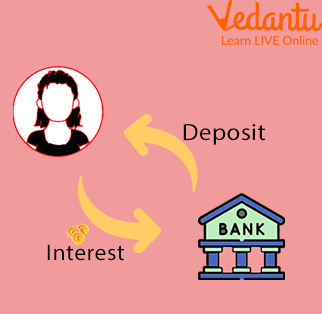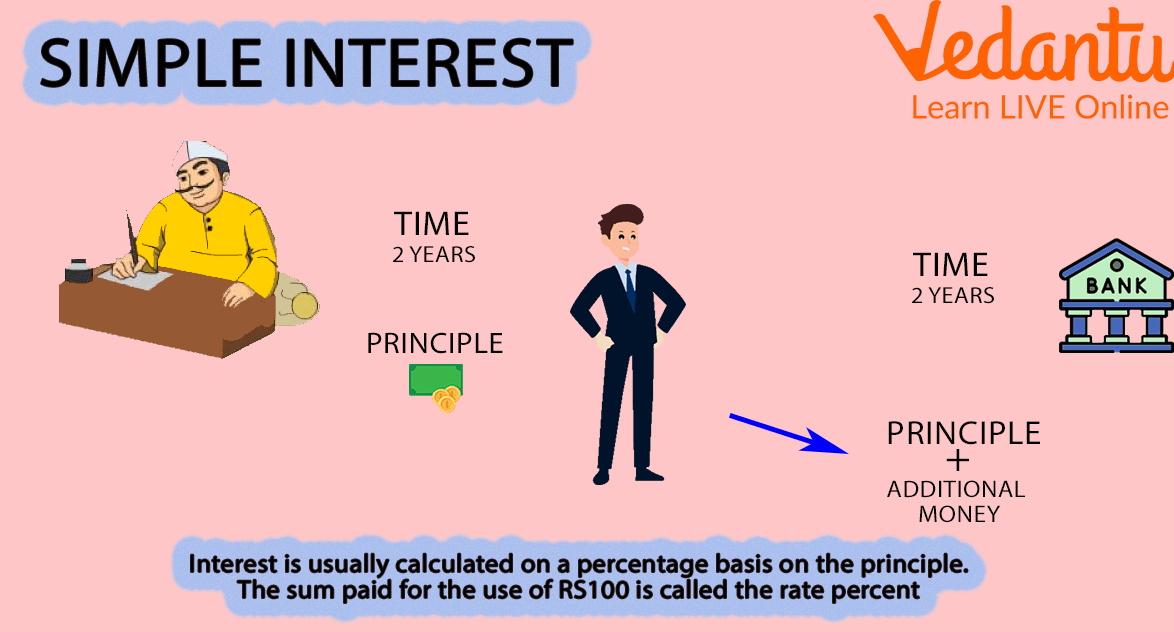Courses
Courses for Kids
Free study material
Free LIVE classes
More

# Simple InterestLIVE
Join Vedantu’s FREE Mastercalss

## Introduction to Simple Interest Problems

When we take a loan from a bank, all the banks charge a rate of interest at which the money is borrowed. Here, we will learn about the simple interest banks put when we lend money from them. But first, we need to understand the term interest, and then we shall learn the Simple Interest. After learning about simple interest concepts, we will go through some simple interest word problems.

## What is Interest?

• The cost of borrowing money is defined as interest.

• Banks, private lenders, etc., all use it.

• It is calculated using a predetermined percentage often chosen or specified by the party giving the loan.

• The Principal Amount refers to the total amount borrowed.

• When we borrow money and subsequently repay it, we pay back the principal sum and the computed additional interest sum.

• A borrower is the one who takes out the loan.

• A lender is an individual who disburses the funds.

• Interest can be of 2 types-

• Simple interestInterest and Deposit Cycle

## Concept of Simple Interest

• Simple interest is an interest rate calculated on the principal amount or the portion of the principal that is still owed.

• It does not take compounding into account.

• Simple interest may be used on a schedule other than annually, such as every month, week, or even every day.

##Simple Interest Representation

## All Formulas of Simple Interest

Below are all formulas of simple interest that have been discussed in detail.

Mathematically,

1. Simple interest (S.I.) = $\dfrac{P \times R \times T}{100}$

Where

P= Principal amount which is to be borrowed

R= Rate of interest fixed by the person who is giving a loan

T= Time in years

It can also be written as

2. $P=\dfrac{S I \times 100}{R \times T}$

3. $R=\dfrac{S I \times 100}{P \times T}$

$T=\dfrac{S I \times 100}{P \times R}$

1. $S.I.=A-P$

Where S.I. is a Simple interest

A is the amount

P is the Principle

With the help of the above two formulas, we can solve simple interest word problems.

Below are the simple interest questions with solutions which can clear the simple interest concepts.

## Simple Interest Questions with Solutions

Q 1. What is the interest paid on Rs. 5430/- for 3 years, at 10%?

Choose the correct options:

1. Rs. 610 /- per annum

2. Rs. 1030 /- per annum

3. Rs. 1629 /- per annum

4. Rs. 500 /- per annum

Ans: Given:

$\mathrm{P}=5430$

$\mathrm{R}=10 \%$

$\mathrm{~T}=3 \text { years } \%$

Simple interest (S.I.) $=\dfrac{P \times R \times T}{100}$ S.I. $=\dfrac{5430 \times 10 \times 3}{100}$

S.I. $=1629 /-$ per annum

Q 2. Richard deposited 5800 and got back an amount of 7000 after a year. Find the simple interest he got.

Ans: Principal (P)= 5800, Amount $(A)= 7000$

S.l. $=\mathrm{A}-\mathrm{P}$

Where S.I. is a Simple interest

$A$ is the amount

$\mathrm{P}$ is the Principle

$=7000-5800= 1200$

Q 3. Seth invested a certain amount of money and got back an amount of Rs 9000. If the bank paid interest of Rs 700, find the amount Sam invested.

Ans: Amount $(A)= 9000$,

Simple Interest (S.I.) $= 700$

S.I. $=\mathrm{A}-\mathrm{P}$

Where S.I. is a Simple interest

$A$ is the amount

$P$ is the Principle

So $P=A-S I$

$=9000-700=7300$

Therefore, Seth invested $7300$.

Q 4. David deposited 20000 for 5 years at a rate of 8% p.a. Find the interest and amount David got.

Ans: Principal $(P)= 20000$,

Time $(T)=5$ years,

Rate $(R)=8 \%$ p.a.

Where S.I. is a Simple interest

$A$ is the amount

$P$ is the Principle

Therefore acc. To this question, $A=P+S I$

$=10000+800= 18000$

Therefore, the amount David got was $18000$.

## Simple Interest Problems for Practice

Q 1. How much time will it take to yield Rs. 8000/- on Rs. 28200/-, if the rate of interest is 8.0%?

A. 2.5 years

B. 4 years

C. 2.3 years

D. 3 years

Ans: 4 years (Option B)

Q 2. If Rs. 5 becomes Rs. 12 in 20 years at simple interest, what will be the rate % p.a.?

Ans: 7%

## Summary

Money cannot be borrowed for free in the real world. You frequently need to take out a loan from a bank to borrow money. In addition to the loan amount, you must pay back additional funds based on the loan amount and the time you borrowed the money. We refer to this as simple interest. In the given article, the topic of simple interest and related word problems are discussed, including the definition and all the formulas.

Last updated date: 26th Sep 2023
Total views: 294k
Views today: 4.94k

## FAQs on Simple Interest

1. What are the Different Kinds of Simple Interest?

Simple interest can be regarded as two classifications when the time is conjectured in terms of days. They are exact and ordinary simple interests. Ordinary simple interest is a SI that takes only 360 days as the equal number of days in a year. While exact simple interest is a SI that considers the exact numbers of days in 365 for a normal year or 366 for a leap year.

In our daily lives, there are 2 types of interest we usually deal with are simple interest and compound interest.

2. What is FVA?

FV also known as final value and A is the amount that is finally calculated by adding simple interest to principal amount (sum of money borrowed). Thus A/FVA= Principal Amount + SI.

3. What is Meant by Investment?

For charging interest on investment, compound Interest can essentially work for you! Investment is an amount where you put money where it can grow, like a bank, financial institution or a business. If we invest your money at a good rate of interest, then it can grow greatly.

4. What are the types of simple interest?

When measured in terms of days, simple interest can be divided into two groups. They are ordinary and straightforward interests. Ordinary simple interest is a SI that only takes 360 days to equal the number of days in a year. Exact simple interest, on the other hand, is a SI that takes exact days in 365 for a normal year or 366 for a leap year.